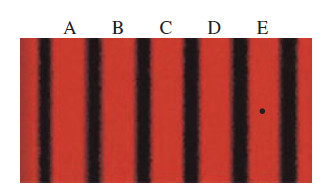# Problem: The figure shows the viewing screen in a double experiment with monochromatic light. Fringe C is the central maximum. A. What will happen to the fringe spacing if the wavelength of the light is decreased?B. What will happen to the fringe spacing if the spacing between the slits is decreased?

###### FREE Expert Solution

The variation of the fringe's width with the slit separation, wavelength, and the distance between the screen and the slit can be expressed as:

$\overline{){\mathbf{\beta }}{\mathbf{=}}\frac{\mathbf{\lambda }\mathbf{D}}{\mathbf{2}\mathbf{D}}}$, where β is the fringe width, λ is the wavelength, D is the distance between the screen and the slit, and 2d is the spacing between the slit.

96% (382 ratings)###### Problem DetailsThe figure shows the viewing screen in a double experiment with monochromatic light. Fringe C is the central maximum.

A. What will happen to the fringe spacing if the wavelength of the light is decreased?

B. What will happen to the fringe spacing if the spacing between the slits is decreased?